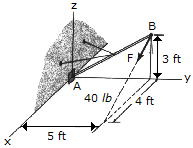# Engineering Mechanics - Force System Resultants - Discussion

### Discussion :: Force System Resultants - General Questions (Q.No.28)

28.Replace the force F, having a magnitude of F = 40 lb and acting at B, by an equivalent force and couple moment at A.

 [A]. F = (32i-24k) lb, M = (-120i+96j-160k) lb-ft [B]. F = (32i-24k) lb, M = 233k lb-ft [C]. F = (32i-24k) lb, M = -72k lb-ft [D]. F = (32i-24k) lb, M = (-120i+160j+40k) lb-ft

Explanation:

No answer description available for this question.